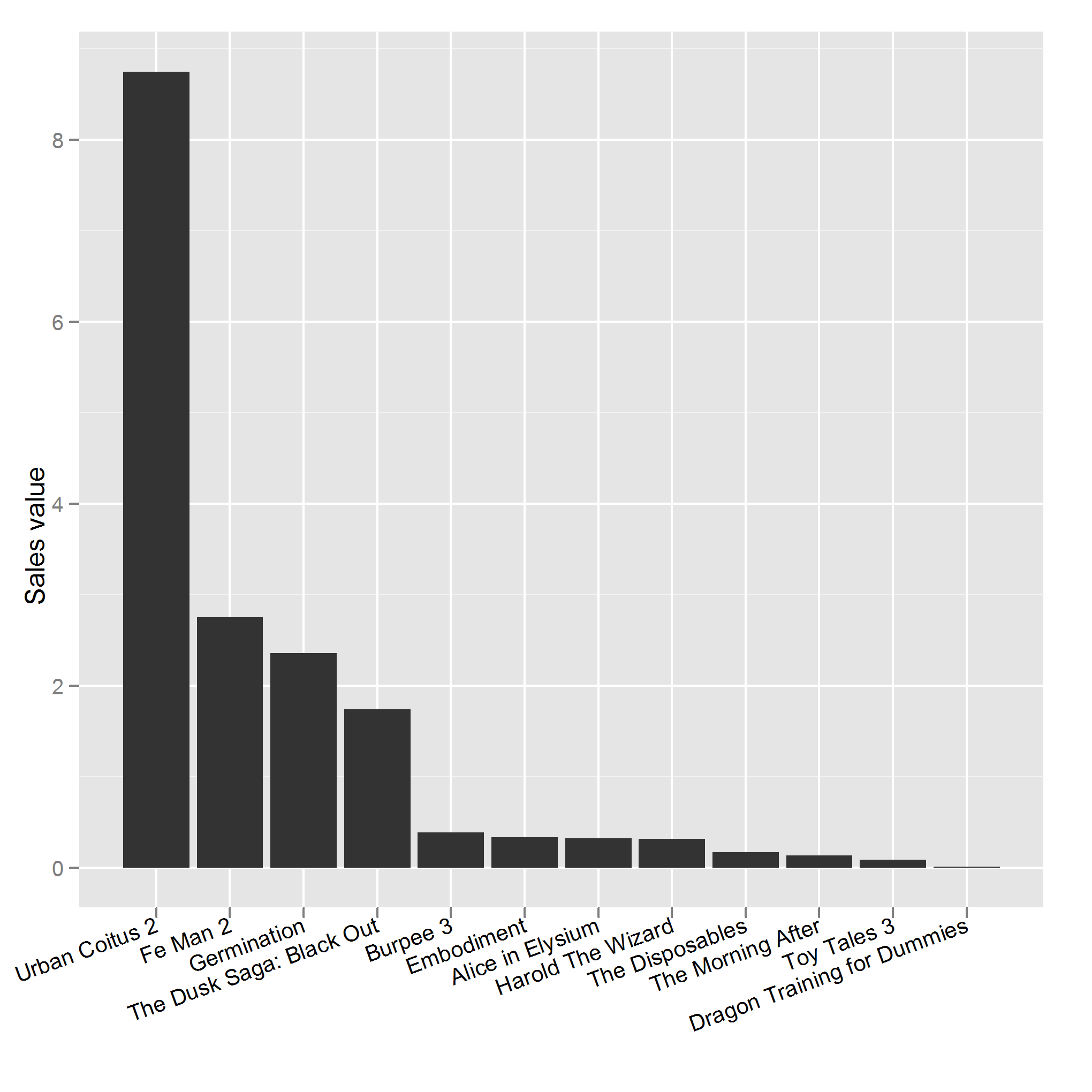Want to share your content on R-bloggers? click here if you have a blog, or here if you don't.

A Pareto plot is an enhanced bar chart. It comes in useful for deciding which bars in your bar chart are important. To see this, take a look at some made up DVD sales data.

```set.seed(1234)
dvd_names <- c("Toy Tales 3", "The Dusk Saga: Black Out", "Urban Coitus 2", "Dragon Training for Dummies", "Germination", "Fe Man 2", "Harold The Wizard", "Embodiment", "Alice in Elysium", "The Disposables", "Burpee 3", "The Morning After")
n_products <- length(dvd_names)
dvd_sales <- data.frame(
product = dvd_names,
value    = rlnorm(n_products) ^ 2
)

library(ggplot2)
bar_chart <- ggplot(dvd_sales, aes(product, value)) +
geom_bar() +
xlab("") +
ylab("Sales value") +
opts(axis.text.x=theme_text(angle=20, hjust=1))
bar_chart
```The “importance” of each bar is given by its height, so we can make the plot easier to interpret by simply sorting the bars from largest to smallest.

```ordered_dvd_sales <- dvd_sales
ordered_dvd_sales\$product <- with(ordered_dvd_sales, reorder(product, -value))
ordered_bar_chart <- bar_chart %+% ordered_dvd_sales
ordered_bar_chart
```Suppose you need to describe what you’ve been selling to your boss. You don’t want to list every DVD because bosses have short attention spans and get confused easily. To make it easy for the boss, you can tell him which films make up 90% of sales. (Or some other percentage — if your catalogue is much bigger then a smaller percentage may be more realistic.)

To find 90% of sales, we need a cumulative total of the sales values. Due to a technicality of `ggplot2`, since bars use a categorical scale but lines require a numeric scale, we also need to convert the x values to be numeric. This function fortifies our dataset with the requisite columns.

```fortify_pareto_data <- function(data, xvar, yvar)
{
for(v in c(xvar, yvar))
{
if(!(v %in% colnames(data)))
{
stop(sQuote(v), " is not a column of the dataset")
}
}

o <- order(data[, yvar], decreasing = TRUE)
data <- data[o, ]
data[, xvar] <- factor(data[, xvar], levels = data[, xvar])

data[, yvar] <- as.numeric(data[, yvar])
data\$.cumulative.y <- cumsum(data[, yvar])

data\$.numeric.x <- as.numeric(data[, xvar])
data
}

#Convert sales to fraction of sales
fractional_dvd_sales <- dvd_sales
total_sales <- sum(fractional_dvd_sales\$value)
fractional_dvd_sales\$value <- fractional_dvd_sales\$value / total_sales

#Now add columns for Pareto plot
fortified_dvd_sales <- fortify_pareto_data(fractional_dvd_sales, "product", "value")

pareto_plot <- bar_chart %+% fortified_dvd_sales +
geom_line(aes(.numeric.x, .cumulative.y)) +
ylab("Percentage of sales") +
scale_y_continuous(formatter = "percent")
pareto_plot
```To see which DVDs constitute 90% of sales, read across from 90% on the y-axis until you hit the cumulative total line. Now read down until you hit the x-axis, and all the DVDs to the left of that point constitute your “important” set. In this case, you’d be telling your boss that 90% of sales come from “Urban Coitus 2″, “Fe Man 2″, “Germination” and “The Dusk Saga: Black Out”.

And there you have it: a Pareto plot. These plots are useful whenever you need to reduce the number of categories of data. As well as these businessy examples, they are great for things like principal component analysis and factor analysis where you need to reduce the number of components/factors.